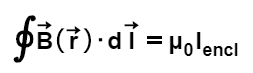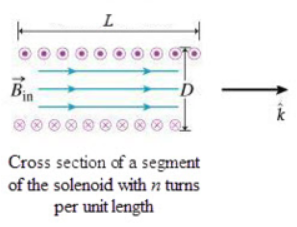# Problem:  Learning Goal: To apply Ampère's law to find the magnetic field inside an infinite solenoid. In this problem we will apply Ampère's law, written to calculate the magnetic field inside a very long solenoid (only a relatively short segment of the solenoid is shown in the pictures). The segment of the solenoid shown in (Figure 1) has length L, diameter D, and n turns per unit length with each carrying current I. It is usual to assume that the component of the current along the z axis is negligible. (This may be assured by winding two layers of closely spaced wires that spiral in opposite directions.) From symmetry considerations it is possible to show that far from the ends of the solenoid, the magnetic field is axial.a) What is Iencl, the current passing through the chosen loop? Express your answer in terms of L (the length of the Amperean loop along the axis of the solenoid) and other variables given in the introduction.b) Find Bin, the z component of the magnetic field inside the solenoid where Ampere's law applies. Express your answer in terms of L, D, n, I, and physical constants such as μ0.

###### FREE Expert Solution

Ampere's law:

$\overline{){\mathbf{\oint }}\stackrel{\mathbf{\to }}{\mathbf{B}}{\mathbf{\text{(}}}\stackrel{\mathbf{\to }}{\mathbf{r}}{\mathbf{\text{)}}}{\mathbf{·}}{\mathbf{d}}\stackrel{\mathbf{\to }}{\mathbf{l}}{\mathbf{=}}{{\mathbf{\mu }}}_{{\mathbf{0}}}{{\mathbf{I}}}_{{\mathbf{encl}}}}$

a)

Enclosed current is given by:

Iencl = NI

86% (426 ratings)###### Problem Details

Learning Goal:

To apply Ampère's law to find the magnetic field inside an infinite solenoid.

In this problem we will apply Ampère's law, writtento calculate the magnetic field inside a very long solenoid (only a relatively short segment of the solenoid is shown in the pictures). The segment of the solenoid shown in (Figure 1) has length L, diameter D, and n turns per unit length with each carrying current I. It is usual to assume that the component of the current along the z axis is negligible. (This may be assured by winding two layers of closely spaced wires that spiral in opposite directions.)

From symmetry considerations it is possible to show that far from the ends of the solenoid, the magnetic field is axial.a) What is Iencl, the current passing through the chosen loop? Express your answer in terms of L (the length of the Amperean loop along the axis of the solenoid) and other variables given in the introduction.

b) Find Bin, the z component of the magnetic field inside the solenoid where Ampere's law applies. Express your answer in terms of L, D, n, I, and physical constants such as μ0.